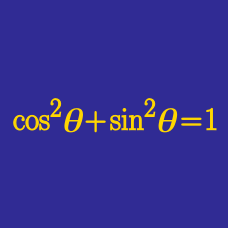Geometry

# Fundamental Trigonometric Identities - Problem Solving

If $\tan \theta = \frac{ 2}{7}$, what is the value of

$\frac{ \cos \theta + 3 \sin \theta } { \cos \theta - 3 \sin \theta}?$

Details and assumptions

You may read up on Trigonometric Functions.

Given that $\sin \theta + \cos \theta = \frac{10}{9}$ and $\sin (2\theta) = \frac{a}{b}$, where $a$ and $b$ are coprime positive integers, what is the value of $a + b$?

If

$\sum_{i=0} ^ {90} \cos ^2 i ^ \circ = \frac {a}{b},$

where $a$ and $b$ are positive coprime integers what is the value of $a+b$?

$P$ is a point on a semicircle with diameter $\overline{AB}=10$. What is the maximum value of $3\overline{AP}+4\overline{BP}?$

What is the value of:

$\left(1-\frac{1}{\cos 72 ^\circ}\right)\left(1+\frac{1}{\sin 18 ^\circ}\right)\left(1-\frac{1}{\sin 72 ^\circ}\right)\left(1+\frac{1}{\cos 18 ^\circ}\right)?$

×